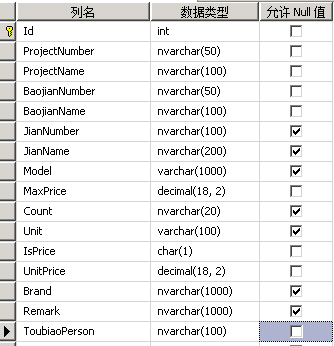1、原始表结构2、查询的sql语句为

select *from(   select *,ROW_NUMBER() OVER (ORDER BY sc desc) as rank    from(    select *,      case when ( 40-(a.p*(case when a.p > 0 then 1 else -0.5 end)))<=30         then 30         else ( 40-(a.p*(case when a.p > 0 then 1 else -0.5 end)))         end as sc     from (    select * from (      select a.ProjectNumber, a.ProjectName, a.BaojianNumber, a.BaojianName, a.ToubiaoPerson,       sum(UnitPrice) as sumPrice,        b.price as avgPrice,        ((sum(UnitPrice)-b.price)/nullif(b.price,0)*100) as p,       sum(case when UnitPrice>b.price then b.price else UnitPrice end )as pprice,       sum(case when UnitPrice>MaxPrice then 1 else 0 end ) as countChao      from ToubiaoDetailTest1 a        join (         select ProjectNumber, ProjectName, BaojianNumber, BaojianName, avg(price) as price         from(          select * from(           select ProjectNumber, ProjectName, BaojianNumber, BaojianName, ToubiaoPerson,             SUM(UnitPrice) as price,            SUM(case when UnitPrice>MaxPrice then 1 else 0 end ) as countChao           from ToubiaoDetailTest1            group BY ProjectNumber, ProjectName, BaojianNumber, BaojianName, ToubiaoPerson          ) tt           where tt.countChao = 0         ) t         group by ProjectNumber, ProjectName, BaojianNumber, BaojianName        ) b          on a.ProjectNumber=b.ProjectNumber and a.ProjectName=b.ProjectName and a.BaojianNumber=b.BaojianNumber and a.BaojianName=b.BaojianName      group by a.BaojianNumber, a.BaojianName, a.ProjectNumber, a.ProjectName, a.ToubiaoPerson, b.price      ) tt      where tt.countChao=0    ) a    ) b  ) t   order by rank

1、根据ProjectNumber, ProjectName, BaojianNumber, BaojianName, ToubiaoPerson分组，查询所有数据的sum(UnitPrice)

其中UnitPrice>MaxPrice的判断是为了逻辑，如果有一条数据满足，则此分组所有的数据不查询。

2、根据ProjectNumber, ProjectName, BaojianNumber, BaojianName 分组，查询所有数据的avg(price)，以上两步主要就是为了查询根据ProjectNumber, ProjectName, BaojianNumber, BaojianName分组的avg(price)值。

3、然后根据逻辑获取相应的值、分数和按照分数排序分页等等操作。

1、建立索引2、索引包含列3、再次优化查询Sql